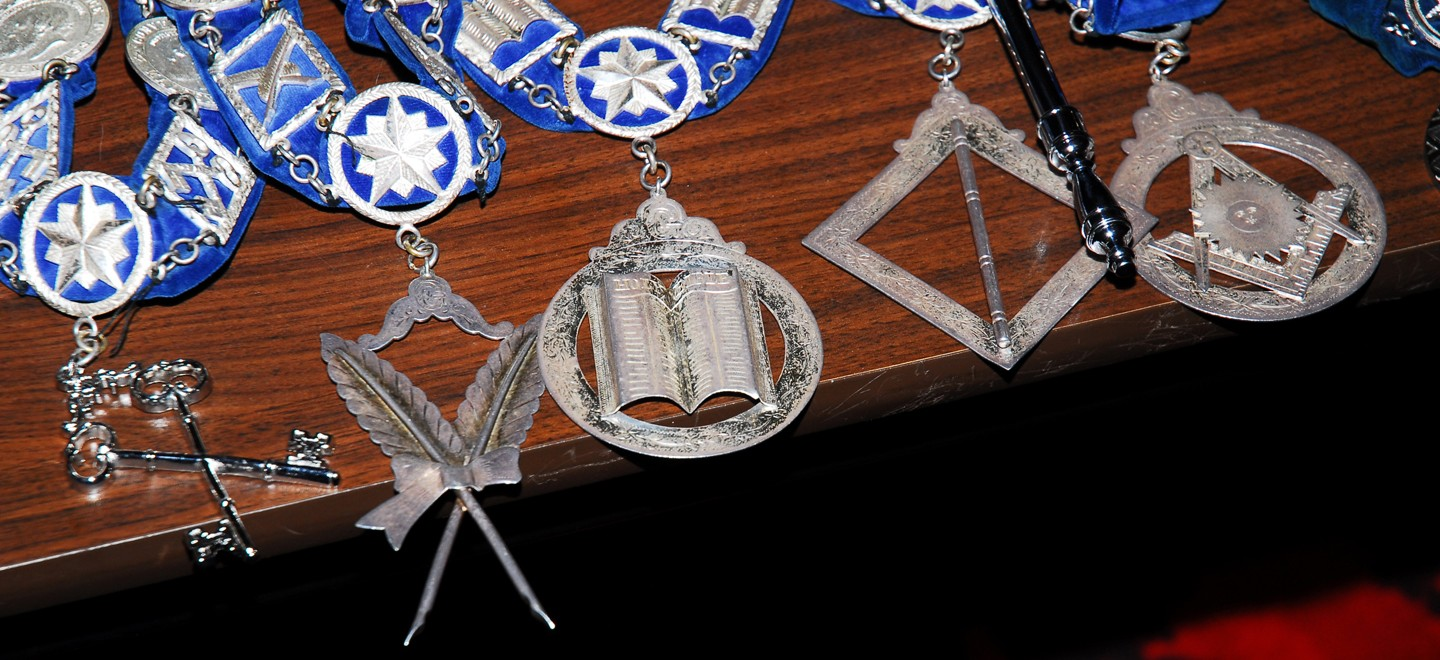Select Page14 + 3 =

9 + 12 =

10 + 9 =

12 + 12 =